Technical Information Site of Power Supply Design

2021.07.14 Simulation

Optimization of PFC Circuits

When modifying the various conditions of a PFC circuit, it may be necessary to adjust the ripple ratio of an inductor current. As one example, a method for adjusting the inductance of an inductor to an appropriate value is shown. The operating mode is assumed to be CCM (continuous current mode).

Circuit Example

The circuit in this example is the "A-4. PFC CCM Vin=200V Iin=2.5A" simulation circuit appearing in the Power Device Solution Circuit/AC-DC PFC list explained in the previous section, "PFC Simulation Circuits Using Solution Circuits" (see Fig. 3). Details of the circuit diagram can be confirmed by clicking the relevant "Schematic Information" icon in the web page list. A direct link to the page is here.

In this example, when conditions in the yellow box in Fig. 3 are changed, the inductance of the inductor is adjusted in order to obtain an appropriate value for the ripple ratio of the inductor current, and the result is confirmed using a simulation.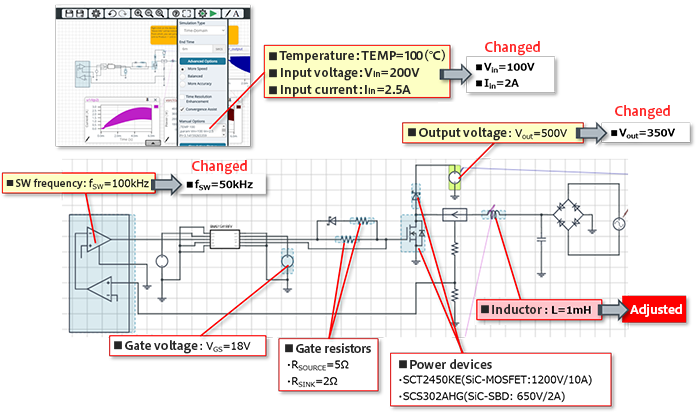Fig. 3: The PFC simulation circuit "A-4. PFC CCM Vin=200V Iin=2.5A" and modified conditions

Fig. 4 shows the results of simulation of the inductor current IL after modification of conditions and before inductance adjustment (default value: 1 mH). In this state, the peak value IL_peak is approximately 3.7 A.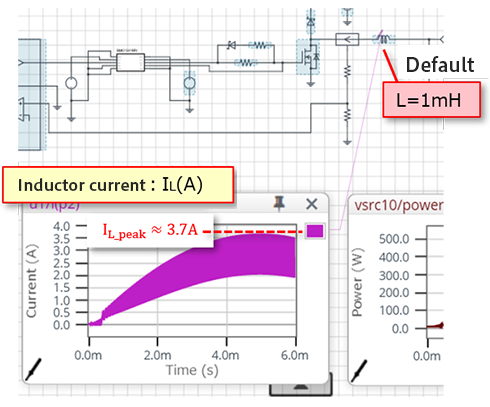Fig 4: Inductor current IL prior to adjustment of the inductance L (default value: 1 mH)

The peak value of the input current is Iin_peak = √2×Iin ≈ 2.82 A, and from these results the ripple ratio M is calculated as follows.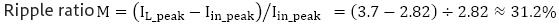The ripple ratio is generally set to less than 30%, and so in this case the inductance L must be adjusted to lower the ripple ratio.

The inductance L is generally computed as follows.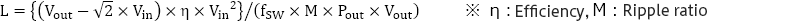When the L value is calculated for η=0.95 and a ripple ratio M of 30% (0.3), the following is obtained.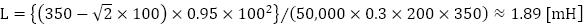When the calculation result is used to change the L value to 2 mH and a simulation is again performed, the inductor current peak is Iin_peak ≈ 3.4 A, as shown in Fig. 5. Hence the ripple ratio is M = (3.4-2.82)/2.82 ≈ 20.6%, and a target value of under 30% is confirmed.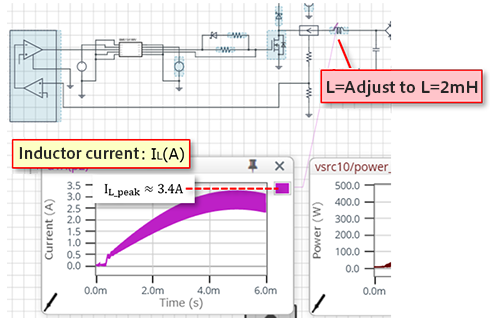Fig.5: Inductor current IL when the inductance L is adjusted to 2 mH

#### Key Points:

・An example is shown in which, when operating conditions are changed in a PFC CCM circuit, the inductance current ripple ratio is corrected by adjusting the inductance.

・An inductance resulting in an appropriate ripple ratio is calculated, and a simulation is performed to verify the result.